The Super Plasticity Behavior between Stress and Parameters in Materials

Journal Name : SunText Review of Material Science

Article Type : Research Article

Authors : Run Xu, Boyong Hur, Hyojun An and Jiaguang Liu

Keywords : Super plasticity; Behavior; Stress; Parameters; Material

Abstract

Three parameters are discussed in this paper. It is found that the superplastic behavior happens in the condition of m=0.6, n=0.09 & K=240MPa. It fits well to formula and m>K>n too in above paper. With decreasing K and n and increasing m the strain will become low to benefit super plastic behavior to happen. ??(K=(?^(1/?) ) ? ) Is a formula to be adopted to super plasticity, here suppose n=1/e. This expresses well the superplastic behavior.

Introduction

The super plasticity is a new approach to gain the biggest super plasticity to satisfy all the demand in material industry. In current the research is developing positively to metals and inorganic materials. The main conditions affect it is micro grains besides low strain rate and temperature [1,2]. In this paper it is modeled that the relationship among stress sensitive exponents m? strength coefficient K and stress exponent n is observed. On the other hand a modeling from elongation convergence is applied to look for the super plasticity. It is found that the super plasticity will happen at 300% elongation from its convergence. It is a new find for super plasticity approach method from pure mathematics. These three parameters are discussed to confirm the relationship among them for us to continue to last find m>K>n . TiAl super plasticity will happen at the conditions of low strain rate (<5×10-4/s) and temperature (>1298K) . So in this paper is adopted that the value in literature above is to model and analyze further study. Overview the parameter is investigated to consider their relationships in order to find the clear factor effectiveness to high strain and elongation. For instance the K-m, K-n and K-stress all they can be found through curve and formulas to look for precision relations of them. The final aim will be known the large elongation condition upon them relations. To try to check the key and subordination effective factor for super plasticity approach and find internal deep relation according to detail classification and analogue is this study ultimate aim and destination.

Modeling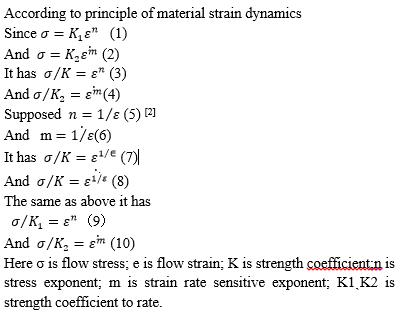Discussion

Since the Three parameters are discussed in this paper. It is found that the superplastic behavior happens in the condition of m=0.6, n=0.09& K=240MPa. It fits well to formula and m>K>n too in above paper. With decreasing K and n and increasing m the strain will become low to benefit super plastic behavior to happen.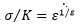is a formula to be adopted to super plasticity, here suppose n=1/e which can be concept from reference . This expresses well the superplastic behavior according to the Figure 1(b). As it is known that there is a summit that arrives 300MPa stress then it curve down to long strain. The lowest one with K=240MPa is near superplastic behavior from the curve that lasts 2000% strain which is dominant super plasticity. The middle one is K=340MPa whose summit arrives 500MPa while the largest is K=440MPa whose summit arrives 600MPa from the Figure 1.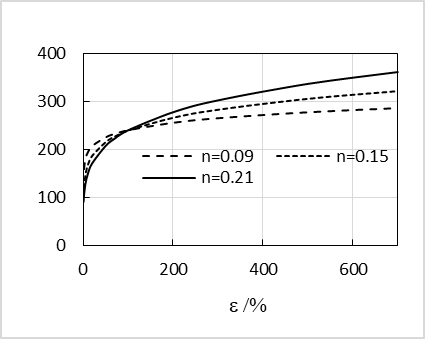(a) n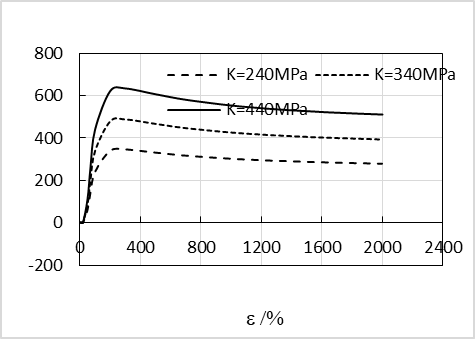(b)K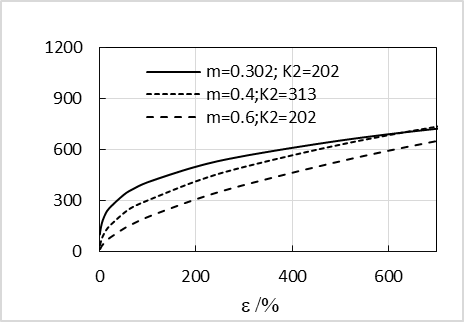(c) m&K2

Figure 1: The relationship between flow stress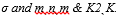So K=240MPa is the best condition for super plasticity which may be less than K=300MPa some. The reasonable region is less than 300MPa as K=240MPa. It is difficult to gain the good plasticity when K=440MPa. It expresses low strain will be attained under this condition. Here K is parameter and ? is stress. From m<K<n relation K is second factor to affect the stress but when it is proposed that n=1/? the result becomes better than original K. so reverse is reasonable to express the phenomenon of super plasticity. The reverse ? is meaning in this study dominantly as literature  expresses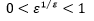that may substitute for n totally and n=1/ ? whose ? is larger than 100% respectively. In terms of this analogue it is can be solved that it is difficult somewhat to explain with theoretical mathematics approach. If the one less than 240MPa of K is adopted the possibility trend is becoming big according to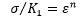etc. whose n is below 0.09 can gain the small stress. But in this study the big n may induce small K to big elongation. When the strain rate m becomes big the small K may be gained too (Figure 1).

As seen in (a~b) the m is weaker parameter totally than the n and m has more increasing trend while n is reasonable with their differences. Here we find the little m and n to cause lower stress that expresses the elongation is good. However the little descending stress is found in (a) that expresses the stability result is created while m causes continuous descending one. Moreover the little stress like 300MPa is good one it explains little stress can make the fine elongation up to 700% strain value. So the n has stable trend than the m here. It is usual that the stability has easily control method than the descending one. So it is concluded that K>n>m is found in this study that is some different result than literature  whose turn is m>K>n in terms of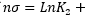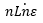. As seen in Figure 1(c) two parameters has been checked and found the optimum one is m=0.6; K2=202 meantime one of m=0.302;K2=202 is high. It expresses that m=0.6 is good one. Overview the big n and m will affect K to small one that causes the small stress directly and gains big strain.

Conclusions

Three parameters are discussed in this paper and it is found that the superplastic behavior happens in the condition of m=0.6, n=0.09& K=240MPa. It fits well to formula and m>K>n too in reference paper. With decreasing K and n and increasing m the strain will become low to benefit super plastic behavior to happen.   is a formula to be adopted to super plasticity, here suppose n=1/e. This will express well the superplastic behavior in materials.

References

1. Zhang K, Chen G, Wang G. Superplasticity in Ceramics J Inorganic Mat. 2003; 18: 707.
2. Run Xu. Convergence Proving of the Theoretical and True Elongation Inequalities by Derivation and Analogy. J Metallic Mat Res. 2020; 3: 15-17.
3. Run Xu. Model for Superplastic Deformation of Ti3Al Alloys. Int J Plant Engineering and Management. 2019; 24: 251-254.
4.  Zhang J, Xu Y, Huang B. Study on deformation Dynamics behaviors of superplasticity in TiAl base alloys. Rare Metal Materials and Engineering. 2014; 43: 1493-1494.